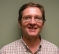## "FFTish" Twiddle FactorsStarted by 5 years ago2 replieslatest reply 5 years ago268 views

I'm dinking with the DFT 128 (sort of FFT) verilog code from open cores.  I've used the 256 point version before.  It has some bugs in it but sort of works out of the box.  With cleanup and pipe-lining the enables it is nice and small.

The 128 point version doesn't work as.  The output isn't right.  It uses a radix 8 followed by a radix 16 stage.  Both designs are nice in that most of the multiplies are fixed and just 4 in the middle are real hardware multipliers.

I want this code so I'm modeling it in Matlab.  I have the two stages, "fft8" and "fft16" working as .m files and they match the Matlab fft.

So next I want to fill a 128 point buffer, operate on it with the fft8 stage, multiply by the twiddle factors, and then operate on that result with the fft16 stage.  There is enough documentation in the core (plus the code) to figure that much out.

However, the twiddle factors in the middle of this I don't know.  The algorithm is called WFTA, or Winograd Fourier transform algorithm.  Anyone familiar enough with this to point me at code or a reference?

Thank you much,

Mark Napier

[ - ]Found it in a book I already had, "Fast Fourier Transform and Convolution Algorithms" by H.J.Nussbaumer

Page 85, Section on the Fast Fourier Transform (isn't the whole dang book on this?)

He considers the DFT of N terms where N = N1 * N2.  Called a 2 factor DFT.  The formula is set up as two summations with the twiddle factors in the middle.

Too long to type up.  I'll post some Matlab code when I have it.

[ - ]